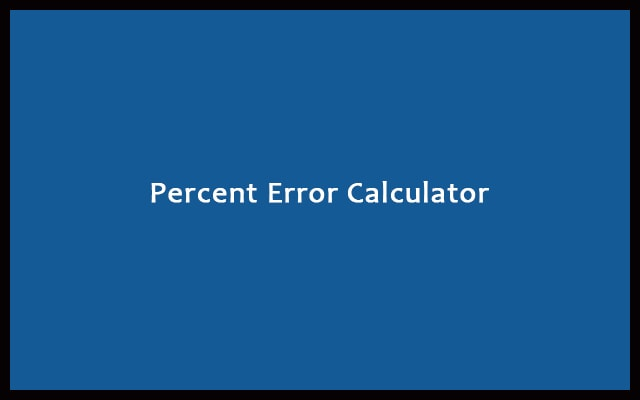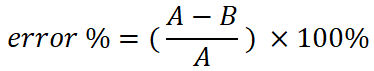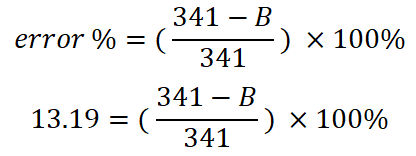# Percent Error Calculator With Formula and Equations

Percent means a fraction of 100. In short, it is part of 100. Similarly, the percent error calculator signifies error percentage. Error part out of 100.

 Enter Approximate/Measured Value: Enter Exact/Actual Value: Percent(%) Error: Percent Error Non Absolute: Absolute Error:
RelatedError is the difference between an exact value and a calculated value. Percentage error is the percentage difference between an exact value and a calculated value. It is essential to find the error percentage. That is why we use the Percent Error Calculator.

With the use of error percent, we will be able to know the deviation from the actual result. It is very helpful in scientific experiments. The least error percent ultimately means more accuracy. If you have done experiments. Whether in Physics or Chemistry. Then you might have found out the error percentage. If the error percent is very high. Then your answer is probably incorrect. In that case, perform the experiment again. Along with more precautions.

Perfect zero percent error is hard to achieve. It is possible when the measured value is equal to the actual value. However, it’s rarely possible. In order to achieve this thing. Your experimental setup must not have any errors. This leads to instrumental error. Every procedure must be carefully executed. Whether you get zero error or not. It is sure that you will come closer to the true value.

## How to Calculate Percent Error?

Percent error calculation is a type of error analysis. To determine the percent error. Therefore, you need two things. Firstly, the exact or true value. Secondly, measured or approximate value. Error is the difference between the true value and the measured value. Percentage error is determined by dividing this difference by true or exact value then multiplying 100. This is the mathematical process that is used by our Percent error calculator.

## Percent Error Formula and Equation

If A is the exact value. If B is the approximate value. Then the error percentage is given by:Example #1

During an experiment. The approximate value was found to be 234. The exact value given is 245. Find the percentage error.

We will use the percent error formula. Here, A (exact value) = 245. B (approximate value) = 234. Now,

error(%) = ((A – B) / A) x 100%

error(%) = ((245 – 234) / 245) x 100%

Therefore, the error percentage is 4.489%.

Example #2

During an experiment. The approximate value was found to be 568. The exact value given is 600. Find the percentage error.

We will use the percent error formula. Here, A (exact value) = 600. B (approximate value) = 568. Now,

error(%) = ((600 – 568) / 600) x 100%

Therefore, the error percentage is 5.333%.

Example #3

For an experiment. Error is found to be 45. The exact value is 341. Determine the error percentage. Also, find the approximate value.

In this case, we already have a difference. It is also called Absolute error. So, directly put in the equation. Also, the exact value is provided. Now,

error(%) = ( error / exact value ) x 100%

error(%) = (45/341) x 100%

Error % is found to be 13.19%.

You can also find the approximate value. Let’s assume the approximate value to be B. Now, we already have the exact value. The exact value or A is equal to 341. Above we have found out the percentage error. Hence, the equation becomesSolving the equation to get the value of B. We finally get B = 269.09.

## Advantages of Percent Error Calculator

• Save time and effort. Use the Percent Error Calculator. To find the percentage error. Our tool takes less than a second. To show the answer. On the other hand, if you go for a manual calculation. Then it will take time. More time if the given values are big.
• While going for manual calculations. No one can guarantee your accuracy. Whether the answer is correct or not. But with the percent error calculator. The accuracy is 100%. Human brains can make a mistake. On the other hand, computers never make errors. It is because they run on pre-defined algorithms. Hence, for every input. It goes through the same process.

#### How to use Percent Error Calculator?

The Percent Error Calculator is an online tool. Hence, enable your Internet connection. On your device open a web browser. Go to the Percent Error Calculator.

There are two input boxes. Enter the exact and approximate values in the respective places. Press the “Calculate” to find percentage error. For the new calculation press “Reset”.